Your data analysis solution

# Log-linear regression (Poisson) in Excel tutorial

2017-10-20

This tutorial will help you set up and interpret a Log-Linear regression, also called Poisson regression in Excel using the XLSTAT software.
Not sure this is the modeling feature you are looking for? Check out this guide

## Dataset for log-linear regression (Poisson regression)

The data correspond to the number of awards earned by students at one high school. To explain the number of awards earned, there are two predictors: the type of program in which the student was enrolled (e.g., vocational, general or academic) and the score on their final exam in math.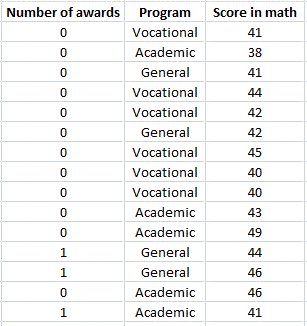Since we have count data, a log-linear regression with a Poisson distribution should be used to explain and/or predict the number of awards earned by a student.

## Setting up a Log-linear regression

After opening XLSTAT, select the XLSTAT / Modeling data / Log-linear regression command, or click on the corresponding button of the Modeling data toolbar.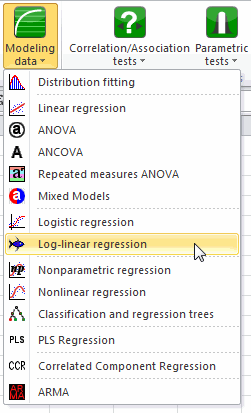Once you've clicked on the button, the dialog box appears.

The data are presented in 200 rows and 3 columns table.  The first column is the response variable and the two others are the explanatory variables.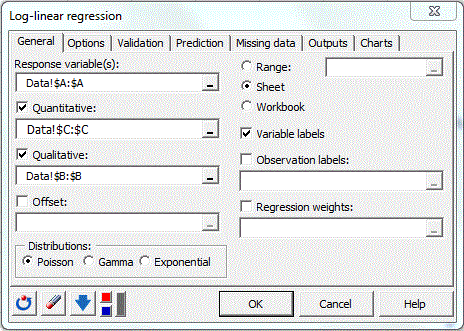In the Options tab, several criteria about the algorithm can be modified. It is also possible to add interactions between the variables. Here we choose to leave all the options to their default values.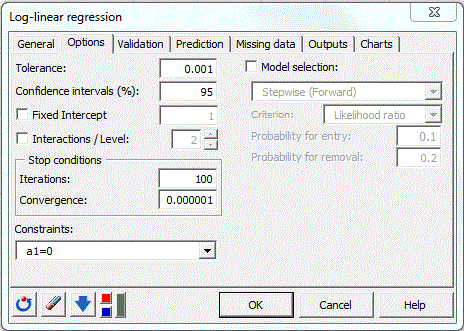The computations begin once you have clicked on OK. The results will then be displayed in a new sheet.

## Interpreting the results

The first results displayed are the statistics for each variable, the dependent variable is represented in blue.

The next table gives several indicators of the quality of the model (or goodness of fit). These results are equivalent to the R2 and to the analysis of variance table in linear regression and ANOVA. The most important value to look at is the probability of Chi-square test on the log ratio. This is equivalent to the Fisher's F test: we try to evaluate if the variables bring significant information by comparing the model as it is defined with a simpler model with only one constant. In this case, as the probability is lower than 0.0001, we can conclude that significant information is brought by the variables.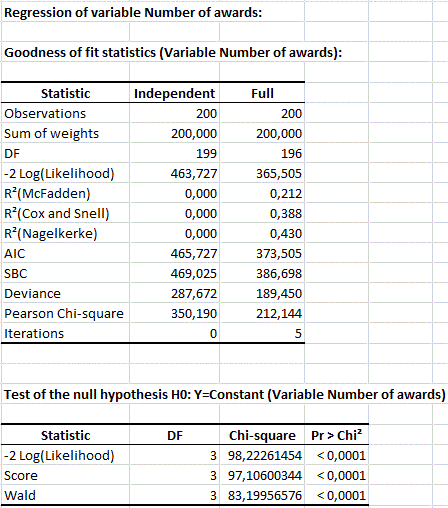The following table gives the estimated value of the coefficients for the fitted model. To evaluate if a variable bring significant information, a statistical test is displayed.  In our case, we note that the parameter of “General Program” does not differ significantly from 0 (at a 5% level).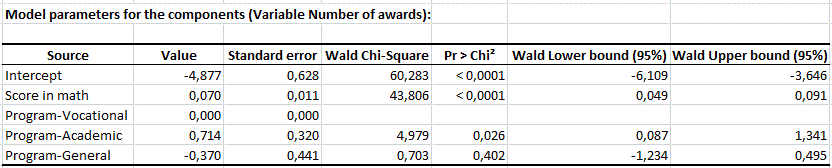The last step is the application of the model on the whole population.

### Contact Us

#### Contact our technical support team: support@xlstat.com

https://cdn.desk.com/
false
desk
Loading
seconds ago
a minute ago
minutes ago
an hour ago
hours ago
a day ago
days ago
about
false
Invalid characters found
/customer/portal/articles/autocomplete
9283